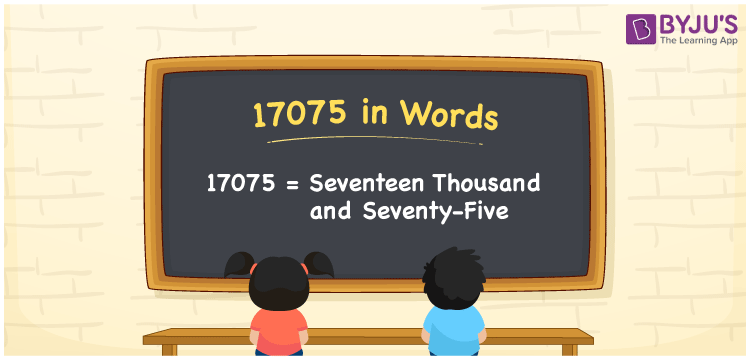# 17075 in Words

17075 in words is written as Seventeen thousand seventy-five. In both the International System of Numerals and the Indian System of Numerals, 17075 is written as Seventeen thousand seventy-five. The number 17075 is a Cardinal Number as it denotes some quantity. For example, “the laptop costs 17075 rupees”.

 17075 in Words Seventeen thousand seventy-five Seventeen thousand seventy-five in Number 17075

## 17075 in English Words

17075 in English words is read as “Seventeen thousand seventy-five”.## How to Write 17075 in Words?

To write 17075 in words, we shall use the place value chart. In the place value chart, put 1 in the ten thousands, 7 in the thousands, 0 in the hundreds, 7 in the tens and 5 in the ones. Let us make a place value chart to write the number 17075 in words.

 Ten Thousands Thousands Hundreds Tens Ones 1 7 0 7 5

Thus, we can write the expanded form as

1 × Ten Thousand + 7 × Thousand + 0 × Hundred + 7 × Ten + 5 × One

= 1 × 10000 + 7 × 1000 + 0 × 100 + 7 × 10 + 5 × 1

= 10000 + 7000 + 0 + 70 + 5

= 17075

= Seventeen thousand seventy-five.

17075 is a natural number, the successor of 17074 and the predecessor of 17076.

17075 in words – Seventeen thousand seventy-five

• Is 17075 an odd number? – Yes
• Is 17075 an even number? – No
• Is 17075 a perfect square number? – No
• Is 17075 a perfect cube number? – No
• Is 17075 a prime number? – No
• Is 17075 a composite number? – Yes

## Frequently Asked Questions on 17075 in Words

Q1

### How to write 17075 in words?

17075 in words is written as Seventeen thousand seventy-five.
Q2

### How to write 17075 in the International and Indian System of Numerals?

In both, the system of numerals, 17075 in words, is written as Seventeen thousand seventy-five.
Q3

### How to write 17075 in a place value chart?

In the place value chart, write 1 in the ten thousands, 7 in the thousands, 0 in the hundreds, 7 in the tens and 5 in the ones, respectively.